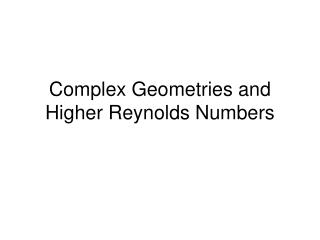DownloadDownload PresentationComplex Geometries and Higher Reynolds Numbers

# Complex Geometries and Higher Reynolds Numbers

Download Presentation## Complex Geometries and Higher Reynolds Numbers

- - - - - - - - - - - - - - - - - - - - - - - - - - - E N D - - - - - - - - - - - - - - - - - - - - - - - - - - -
##### Presentation Transcript

1. Complex Geometries and Higher Reynolds Numbers

2. Creating obstacle files • Current LB2D_Prime naming convention: • sizexXsizey.bmp • Current format: • ‘True color’ 24 bit

3. Scaling to real world • Choose a Reynolds number • Choose a combination of slit width and viscosity to give maximum velocity < ≈0.1 lu ts-1; smaller is better • Maximum velocity 3/2 of average for Poiseuille flow in slit • Best to use t = 1 for simple bounceback boundaries: yields kinematic viscosity of 1/6 lu2 ts-1 • Solve for the gravitational acceleration needed to drive the flow by rearranging Poiseuille equation

4. Entry Length Effects • r is the 'radius' -- the distance from the center to the point of interest • a is the half-width (d/2) • u is the velocity at the point of interest and uavg is the average velocity Tritton DJ (1988) Physical Fluid Dynamics, 2nd Ed. Oxford University Press, Oxford New York x /(d Re) is dimensionless distance down the pipe. When this distance is infinite, Poiseuille flow is fully developed. If Re = 1, Poiseuille flow is well-developed in a short distance down the pipe: x/d = 1 →x = d = 2a (just 2 half-widths down the pipe). As the Reynolds number increases, this distance can become quite large. If x = 1 m with Re = 103 in a 10 cm pipe, x/(d Re) = 1 m /(10-1 m 103) = 10-2 and Poiseuille flow will not be fully developed even 1 m from the inlet.

5. Entry Length Effects Tritton DJ (1988) Physical Fluid Dynamics, 2nd Ed. Oxford University Press, Oxford New York

6. Velocity BCs • Simple compressible fluid model • Identical constant velocities at each end of the domain: • mass of fluid will change with time • Flow must be accompanied by a pressure gradient and hence the pressure must be lower at the outlet • The pressure and density are related through an ideal gas law of the form P = r/3 in this model • Densities at the input and output must be different • If the velocity boundaries on each end of the domain are equal, mass will accumulate in the system because the mass flux of fluid in (vinrin) will exceed the out flux (voutrout) • Problem increases in severity as the pressure difference increases • Incompressible model of Zou and He (1997), pressure boundaries, or gravity-driven flow can be used to avoid this complication

7. Flow Past a Cylinder • The drag force FD is defined in terms of the drag coefficient CD as • Gravitational Force

8. http://scienceworld.wolfram.com/physics/CylinderDrag.html Tritton DJ (1988) Physical Fluid Dynamics, 2nd Ed. Oxford University Press, Oxford New York

9. Re = 0.16

10. Re = 41 Taneda S (1956)

11. von Kármán Vortex Street, Re = 105 h l Photo by S. Taneda. S. Taneda and the Society for Science on Form, Japan